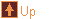Tensegrity Models 15 images tree :: Geometry > Tensegrity Models
In This Album:

 Image List Thumbnail Page
These tensegrity models have been made by connecting together edge units to form twisted polyhedra. The edge units have a notch at each end, and a loop of cord running along the length. They connect by slipping the cord of one edge into the notch of another. Each unit connects with four others: one at each notch and two along the cord. The basic idea is explained nicely by George Hart in his page on Soda Straw Tensegrity Structures.

I have built my units from two barbecue sticks bound by wire near each end, which gives the notches. The cord is either a loop of string, or a length of elastic knotted at each end and sliped through the notches.

The process of twisting transforms an equal edge polyhdron into an equal edge dual form. In the case of the regular and quasi-regular polyhedra that dual has the same shape as the "normal" dual (by polar reciprocation). Not all equal edge polyhedra have an equal edge twisted dual.

The regular and quasi-regular polyhedra are also linked with their twisting mid-point by a jitterbug-like transformation, as described in Polyhedral Twisters.

Twisted polyhedra are also popular as a base for wooden puzzles. A lot of these puzzles can be seen at Puzzle World.

 thumbnails 1/1
 OctahedronMed  Lrg
 Octahedron / CubeMed  Lrg
 CubeMed  Lrg
 CuboctahedronMed  Lrg
 Cuboctahedron / Rhombic Dodecahedron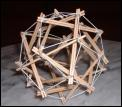Med  Lrg
 Rhombic DodecahedronMed  Lrg
 Rhombic DodecahedronMed  Lrg
 IcosahedronMed  Lrg
 Icosahedron / DodecahedronMed  Lrg
 Dodecahedron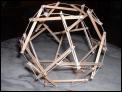Med  Lrg
 Small Stellated Dodecahedron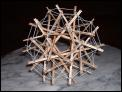Med  Lrg
 Small Stellated DodecahedronMed  Lrg
 Small Stellated DodecahedronMed  Lrg
 Stella Octangula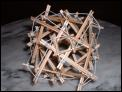Med  Lrg
 Stella Octangula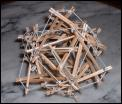Med  Lrg
 1